## Selina Concise Mathematics Class 10 ICSE Solutions Chapter 21 Trigonometrical Identities (Including Trigonometrical Ratios of Complementary Angles and Use of Four Figure Trigonometrical Tables) Ex 21B

These Solutions are part of Selina Concise Mathematics Class 10 ICSE Solutions. Here we have given Selina Concise Mathematics Class 10 ICSE Solutions Chapter 21 Trigonometrical Identities Ex 21B.

Other Exercises

Question 1.
Prove that:Solution:Solution: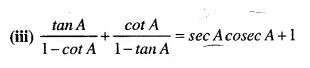Solution:Solution:Solution: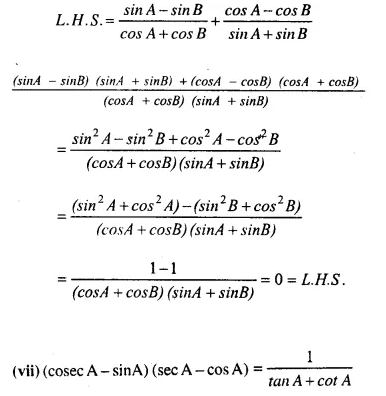Solution: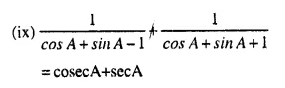Solution: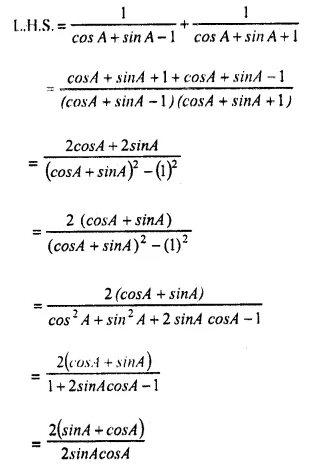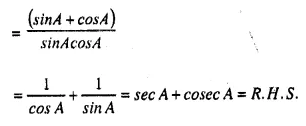Question 2.
If xcosA + ysinA = m and xsinA-ycosA = n, then prove that: x2+y2 = m2 + n2
Solution:
x cos A + y sin A = m    …(i)
x sin A – y cos A = n     ….(ii)
squaring (i) and (ii)
x2 cos2 A + y2 sin2 A + 2 xy cosA sinA = m2
x2 sin2 A + y2 cos2 A – 2 xy cos A sin A = n2
x2 (sin2 A + cos2 A) + y2 (sin2 A + cos2 A) = m2+n2
∴ x2+y2 = m2 + n2(∵ sin2A + cos2A= 1)
Hence proved.

Question 3.
If m = a sec A +b tan A and n=atanA + bsecA, then prove that: m2-n2 = a2-b2
Solution:
m = asec A + btan A         ……(i)
n = a tan A + b sec A       …..(ii)
squaring (i) and (ii)
m2 = a2 sec2 A + b2 tan2 A + 2ab sec A tan A
n2 = a2 tan2 A + b2 sec2 A + 2 ab tan A sec A
Subtracting, we get
m2 – n2 = a2 (sec2 A – tan2 A) + b2 (tan2 A – sec2 A)
= a2x 1 +b2(-1) = a2-b2 ( ∵ sec2A-tan2A= 1)  .
Henceproved

Question 4.
If x = r sin A cos B, y = r sin A sin B and z = r cos A, then prove that: x2 + y2 + z2 = r.
Solution:
x = r sin A cos B      ….(i)
y = r sin A sin B      ….(ii)
z = r cosA               …….(iii)
Squaring, (i), (ii) & (iii)
x2=r2 sin2 A cos2 B,
y2 = r2sin2Asin2B,
z2 = r2cos2A
x2+y2 + z2=r2 (sin2A cos2E + sin2 A sin2 B+cos2A)
= r[sin2A (cos2 B + sin2B) + cos2A]
= r [sin2 A x 1 + cos2 A]
= r2 [sin2 A + cos2 A] = r2 x 1  = r2        ( ∵ sin2 A + cos2 A = 1)
Hence proved.

Question 5.
If sin A + cos A = m and sec A + cosec A=n, show that n (m2-1) = 2m
Solution:Question 6.
If x = r cos A cos B, y = r cos A sin B and z = r sin A, show that x2 + y2 + z2 = r2
Solution:
x = r cosAcosB              ….(i)
y = r cosAsinB             ….(ii)
z = r sinA                 ….(iii)
Squaring (i), (ii), (iii)
x2 = r2 cos2 A cos2 B, y2 = r2 cos2 A sin2B
z2 = r2sin2A
x2 + y2 + z2 = r2 (cos2 A cos2B + cos2 A sin2 B + sin2 A)
= r2 [cos2 A (cos2 B + sin2B) + sin2 A]
= r2[cos2Ax 1+sin2A]
= r2 (1) = r2    `Hence proved.

Question 7.Solution: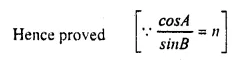P.Q.Solution: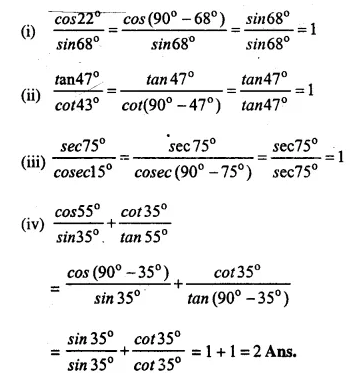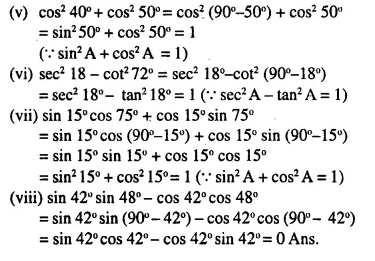P.Q.
Evaluate: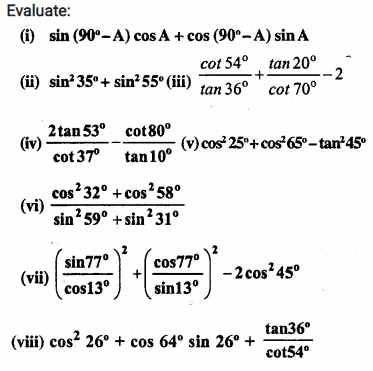Solution: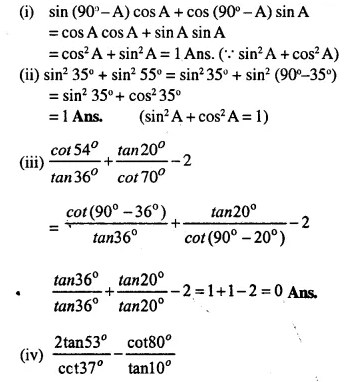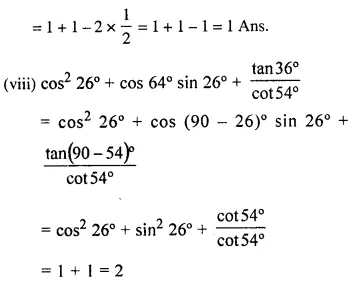Hope given Selina Concise Mathematics Class 10 ICSE Solutions Chapter 21 Trigonometrical Identities Ex 21B are helpful to complete your math homework.

If you have any doubts, please comment below. Learn Insta try to provide online math tutoring for you.